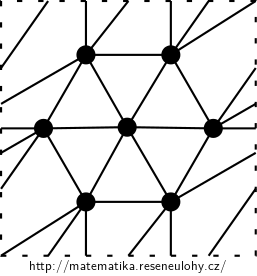## Complete graph on the torus

Which complete graphs $$K_n$$ can be embedded, i.e. drawn without crossing edges, into the torus?

• #### Hint

Use Euler’s formula for the torus.

Alternatively, use Heawood’s formula.

• #### Solution

Solution via Euler’s formula:

For $$K_n$$: $$| V | (| V | -1) = 2 | E |$$ and $$| F | \leq \frac {2} {3} | E |$$. Euler’s formula for surfaces of higher genus is: $$| V | + | F | = | E | + 2 - g$$. The torus has $$g = 0$$. By substituting we get: $\begin {eqnarray*} |V| + |F| &=& |E| + 2 - g \\ |V| + \frac{2}{3} |E|&\geq& |E| \\ 6|V| + 4|E| &\geq& 6|E| \\ 6|V| - 2|E| &\geq& 0\\ 6n -n^2 + n &\geq& 0 \\ n^2 -7n &\leq& 0 \\ (n-7) &\leq& 0 \\ \end {eqnarray*}$

Solution via Heawood’s formula:

The torus has Euler characteristic $$\chi = 0$$ and the Heawood bound yields $$h (\chi) = \lfloor (7 + \sqrt {49-24{\cdot} 0}) / 2 \rfloor = 7$$

In both cases, the upper bound is 7, i.e. graphs larger than $$K_7$$ cannot be embedded. You can draw $$K_7$$, for example, starting by the cycle $$C_6$$ so that we can add a universal vertex inside, as in the picture..The largest complete graph that can be drawn on the torus is $$K_7$$.